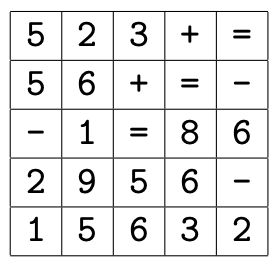시간 제한 메모리 제한 제출 정답 맞은 사람 정답 비율
2 초 512 MB 0 0 0 0.000%

## 문제

A m × n table is filled with symbols from the set S = {0, 1, 2, . . . , 9, +, -, *, (, )}, satisfying 3 ≤ m, n ≤ 8. The symbols 0, 1, 2,. . ., 9 represent digits, and +, -, and * represent addition, subtraction, and multiplication respectively. The notation ( stands for opening parenthesis and ) for closing one.

You have to trace all possible paths starting from any cell in the first row to any cell in the last row. Any path should yield a valid numeric equation that contains exactly one equal sign. You are allowed to move only in four primary directions: up, down, left, and right. No diagonal moves are allowed. The numerical contents of adjacent cells along the path may form a number, but that number should not exceed more than four digits or contain leading zeros (except for 0, which should consist of a single digit of zero). You cannot use any cellular content more than once in an equation. Symbols + or - cannot be used for positive or negative signs.

The figure below is a tabular representation of the sample input.You are required to print all possible valid equations.

## 입력

The input file comprises of several blocks. Each block begins with a line containing two integers for m (the number of rows) and n (the number of columns), separated by one or more spaces. The rest of the block is an m × n table filled with elements from the set S separated by one or more spaces.

Blocks are separated by a blank line. The input file is terminated by two zeros on a line by itself following the last block.

## 출력

For each block, first print its number (Table 1, Table 2, etc.) and then all possible equations.

Each line should represent a single equation. Equations may be printed in any order, but no equation is allowed to appear more than once even if the equation can be obtained by more than one paths. In this problem, equations are identical if and only if they match literally.

If no equation could be obtained from a block simply print “No Solution” instead of equations.

Print a blank line after each block. No extra spaces or blank lines should be printed.

## 예제 입력 1

5 5
5 2 3 + =
5 6 + = -
- 1 = 8 6
2 9 5 6 -
1 5 6 3 2
0 0


## 예제 출력 1

Table 1
23+61=86-2
55-1=56-2